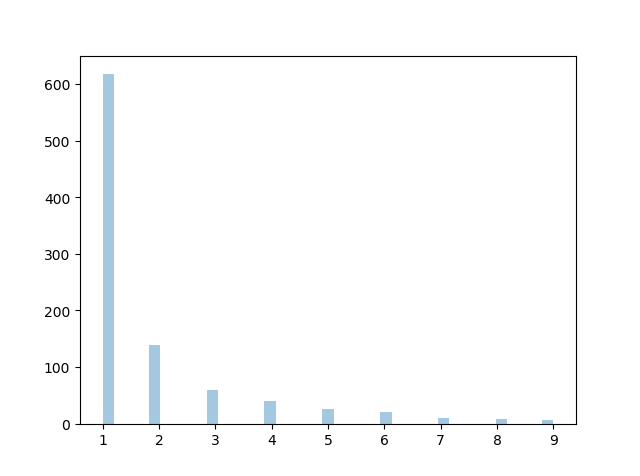# Zipf Distribution

Zipf distributions are used to sample data based on zipf's law.

Zipf's Law: In a collection, the nth common term is 1/n times of the most common term. E.g. the 5th most common word in English occurs nearly 1/5 times as often as the most common word.

It has two parameters:

`a` - distribution parameter.

`size` - The shape of the returned array.

### Example

Draw out a sample for zipf distribution with distribution parameter 2 with size 2x3:

from numpy import random

x = random.zipf(a=2, size=(2, 3))

print(x)
Try it Yourself »

## Visualization of Zipf Distribution

Sample 1000 points but plotting only ones with value < 10 for more meaningful chart.

### Example

from numpy import random
import matplotlib.pyplot as plt
import seaborn as sns

x = random.zipf(a=2, size=1000)
sns.distplot(x[x<10], kde=False)

plt.show()

### ResultTry it Yourself »

W3Schools is optimized for learning and training. Examples might be simplified to improve reading and learning. Tutorials, references, and examples are constantly reviewed to avoid errors, but we cannot warrant full correctness of all content. While using W3Schools, you agree to have read and accepted our terms of use, cookie and privacy policy.